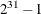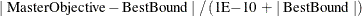### DECOMP Statement

DECOMP <decomp-options> ;

The DECOMP statement controls the overall decomposition algorithm.

Table 13.3 summarizes the decomp-options available in the DECOMP statement. These options control the overall decomposition algorithm process flow during the solution of an LP or a MILP. (As indicated, you can specify the data set options only in the OPTLP or OPTMILP procedure, and you can specify some control options only for a MILP.)

Table 13.3: Options in the DECOMP Statement

Description

decomp-option

Data Set Options (OPTLP and OPTMILP procedures only)

Specifies the blocks input data set

Control Options

Specifies the stopping criterion based on an absolute objective gap

Specifies the frequency of removing ineffective columns from the master LP

Specifies whether to initialize the columns by solving each block with the original cost vector

Specifies the level of detail of solution progress to print in the log

Specifies the maximum number of blocks to allow

Specifies the maximum number of new columns to allow into the master each pass

Specifies the maximum amount of time spent in the decomposition algorithm

Specifies the decomposition algorithm method

Specifies the stopping criterion based on relative objective gap

Control Options (MILP only)

Specifies how frequently to print the continuous iteration log

Specifies whether the master problem is solved as a MILP with the current set of columns at the beginning of phase II

Specifies whether the master problem is solved as a MILP with the current set of columns at the end of phase II

Specifies the frequency of solving the master problem as a MILP with the current set of columns

Specifies the maximum number of outer iterations for the decomposition algorithm

The following list describes the decomp-options in detail.

ABSOBJGAP=number

specifies a stopping criterion for the continuous bound of the decomposition. When the absolute difference between the master objective and the best dual bound falls below the value of number, the decomposition algorithm stops adding columns. The value of number can be any nonnegative number. The default value is the value of the OPTTOL= main solver option.

BLOCKS=SAS-data-set

specifies (for OPTLP and OPTMILP procedures only) the input data set that contains block definitions to be used by the decomposition algorithm if METHOD=USER. See the section The BLOCKS= Data Set in PROC OPTMILP and PROC OPTLP for more information. To specify blocks in PROC OPTMODEL, use the .block constraint suffix instead (see the section The .block Constraint Suffix in PROC OPTMODEL).

COMPRESSFREQ=number

removes ineffective columns from the master LP after every number of iterations. The frequency, number, is an integer between 0 and the largest four-byte signed integer, which is. The default value is 0.

INITVARS=number | string

specifies whether to initialize the columns by using the original cost vector to solve each block.

Table 13.4 describes the valid values of the INITVARS= option.

Table 13.4: Values for INITVARS= Option

number

string

Description

0

OFF

Disables initializing the columns by using the original cost vector to solve each block.

1

ON

Enables initializing the columns by using the original cost vector to solve each block.

The default is ON.

LOGFREQ=number

specifies (for MILP problems only) how often to print information in the continuous iteration log. The value of number can be any nonnegative number up to the largest four-byte signed integer, which is. The default value of number is 10. If number is set to 0, then the iteration log is disabled. If number is positive, then an entry is made in the log at the first iteration, at the last iteration, and at intervals that are dictated by the value of number. An entry is also made each time a better integer solution or improved bound is found.

LOGLEVEL=number | string

controls the amount of information that is displayed in the SAS log by the decomposition algorithm. Table 13.5 and Table 13.6 provide the valid values for this option and a description of what is displayed in the log when an LP and a MILP, respectively, is solved.

Table 13.5: Values for LOGLEVEL= Option for an LP

number

string

Description

–1

AUTOMATIC

Prints the continuous iteration log at the interval dictated by the LOGFREQ= main solver option.

0

NONE

Turns off printing of all of the decomposition algorithm messages to the SAS log.

1

BASIC

Prints the continuous iteration log at the interval dictated by the LOGFREQ= main solver option.

2

MODERATE

Prints the continuous iteration log and summary information for each iteration at the interval dictated by the LOGFREQ= main solver option.

3

AGGRESSIVE

Prints the continuous iteration log and detailed information for each iteration at the interval dictated by the LOGFREQ= main solver option.

Table 13.6: Values for LOGLEVEL= Option for a MILP

number

string

Description

–1

AUTOMATIC

Prints the continuous iteration log for the root node at the interval dictated by the LOGFREQ= option in the DECOMP statement. Prints the branch-and-bound node log at the interval dictated by the LOGFREQ= main solver option.

0

NONE

Turns off printing of all of the decomposition algorithm messages to the SAS log.

1

BASIC

Prints the continuous iteration log for each branch-and-bound node at the interval dictated by the LOGFREQ= option in the DECOMP statement.

2

MODERATE

Prints the continuous iteration log and summary information for each iteration of each branch-and-bound node at the interval dictated by the LOGFREQ= option in the DECOMP statement.

3

AGGRESSIVE

Prints the continuous iteration log and detailed information for each iteration of each branch-and-bound node at the interval dictated by the LOGFREQ= option in the DECOMP statement.

The default is AUTOMATIC for both LPs and MILPs.

MASTER_IP_BEG=number | string

specifies (for MILP problems only) whether the master problem is solved as a MILP with the current set of columns at the beginning of phase II. Table 13.7 describes the valid values of the MASTER_IP_BEG= option.

Table 13.7: Values for MASTER_IP_BEG= Option

number

string

Description

0

OFF

Disables solving the master as a MILP at the beginning of phase II.

1

ON

Enables solving the master as a MILP at the beginning of phase II.

The default is ON in the root node and 0 elsewhere.

MASTER_IP_END=number | string

specifies (for MILP problems only) whether the master problem is solved as a MILP with the current set of columns at the end of phase II. Table 13.8 describes the valid values of the MASTER_IP_END= option.

Table 13.8: Values for MASTER_IP_END= Option

number

string

Description

0

OFF

Disables solving the master as a MILP at the end of phase II.

1

ON

Enables solving the master as a MILP at the end of phase II.

The default is ON in the root node and 0 elsewhere.

MASTER_IP_FREQ=number

solves the master problem (for MILP problems only) as a MILP with the current set of columns after every number iterations. The frequency, number, is an integer between 0 and the largest four-byte signed integer, which is. The default is 10 in the root node and 0 elsewhere.

MAXBLOCKS=number

specifies the maximum number of blocks to allow. If the defined number of blocks exceeds number, the algorithm creates superblocks using a very simple round-robin scheme. The value number can be any integer between 1 and the largest four-byte signed integer, which is. The default value of number is.

MAXCOLSPASS=number

specifies the maximum number of new columns to allow into the master at each pass. This option is disabled on the initial pass if INITVARS=1. The value number can be any integer between 1 and the largest four-byte signed integer, which is. The default value of number is.

MAXITER=number

specifies (for MILP problems only) the maximum number of outer iterations for the decomposition algorithm. The value number can be any integer between 1 and the largest four-byte signed integer, which is. If you do not specify this option, the procedure does not stop based on the number of iterations performed.

MAXTIME=number

specifies an upper limit of number seconds of time for the decomposition algorithm. The value of the TIMETYPE= main solver option determines the type of units used. If you do not specify this option, the procedure does not stop based on the amount of time elapsed. The value of number can be any positive number; the default value is the positive number that has the largest absolute value that can be represented in your operating environment.

METHOD=string

specifies the decomposition algorithm method as shown in Table 13.9.

Table 13.9: Values for METHOD= Option

string

Description

USER

The user defines which rows belong to which blocks (subproblems). In PROC OPTMODEL, use the .block constraint suffix. In PROC OPTLP and PROC OPTMILP, use the BLOCKS= data set instead.

NETWORK

The algorithm attempts to find an embedded network similar to what is described in The Network Simplex Algorithm. The weakly connected components of this network are used as the blocks.

AUTO

The algorithm attempts to find a block structure in the constraint matrix. For the current release, METHOD=AUTO finds block-diagonal structure only (not block-angular structures); unless your problem separates into completely independent problems with no linking constraints, this method finds only one block and hence is equivalent to calling the MILP solver directly.

The default is USER if blocks are defined and NETWORK otherwise.

RELOBJGAP=number

specifies the relative objective gap as a stopping criterion. The relative objective gap is based on the master objective (MasterObjective) and the best dual bound (BestBound); it is equal toWhen this value becomes smaller than the specified gap size number, the decomposition algorithm stops adding columns. The value of number can be any nonnegative number. For LP, the default value is 0; for MILP, the default value is 1e-4.